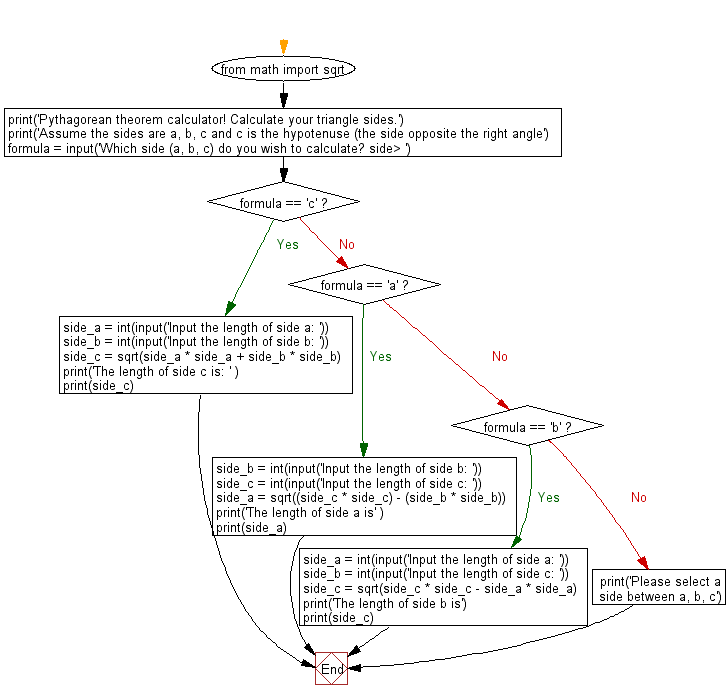﻿ Python Math: Create a Pythagorean theorem calculator - w3resource# Python Math: Create a Pythagorean theorem calculator

## Python Math: Exercise-68 with Solution

Write a Python program to create a Pythagorean theorem calculator.

Note : In mathematics, the Pythagorean theorem, also known as Pythagoras' theorem, is a fundamental relation in Euclidean geometry among the three sides of a right triangle. It states that the square of the hypotenuse (the side opposite the right angle) is equal to the sum of the squares of the other two sides.

Sample Solution:-

Python Code:

``````from math import sqrt

print('Pythagorean theorem calculator! Calculate your triangle sides.')
print('Assume the sides are a, b, c and c is the hypotenuse (the side opposite the right angle')
formula = input('Which side (a, b, c) do you wish to calculate? side> ')

if formula == 'c':
side_a = int(input('Input the length of side a: '))
side_b = int(input('Input the length of side b: '))

side_c = sqrt(side_a * side_a + side_b * side_b)

print('The length of side c is: ' )
print(side_c)

elif formula == 'a':
side_b = int(input('Input the length of side b: '))
side_c = int(input('Input the length of side c: '))

side_a = sqrt((side_c * side_c) - (side_b * side_b))

print('The length of side a is' )
print(side_a)

elif formula == 'b':
side_a = int(input('Input the length of side a: '))
side_b = int(input('Input the length of side c: '))

side_c = sqrt(side_c * side_c - side_a * side_a)

print('The length of side b is')
print(side_c)

else:
print('Please select a side between a, b, c')
```
```

Sample Output:

```Pythagorean theorem calculator! Calculate your triangle sides.
Assume the sides are a, b, c and c is the hypotenuse (the side opposite the right angle
Which side (a, b, c) do you wish to calculate? side>a
Input the length of side b:10
Input the length of side c:15
The length of side a is
11.180339887498949
```

Flowchart:## Visualize Python code execution:

The following tool visualize what the computer is doing step-by-step as it executes the said program:

Python Code Editor:

Have another way to solve this solution? Contribute your code (and comments) through Disqus.

What is the difficulty level of this exercise?

Test your Programming skills with w3resource's quiz.

﻿

## Python: Tips of the Day

Clamps num within the inclusive range specified by the boundary values x and y:

Example:

```def tips_clamp_num(num,x,y):
return max(min(num, max(x, y)), min(x, y))
print(tips_clamp_num(2, 4, 6))
print(tips_clamp_num(1, -1, -6))
```

Output:

```4
-1
```